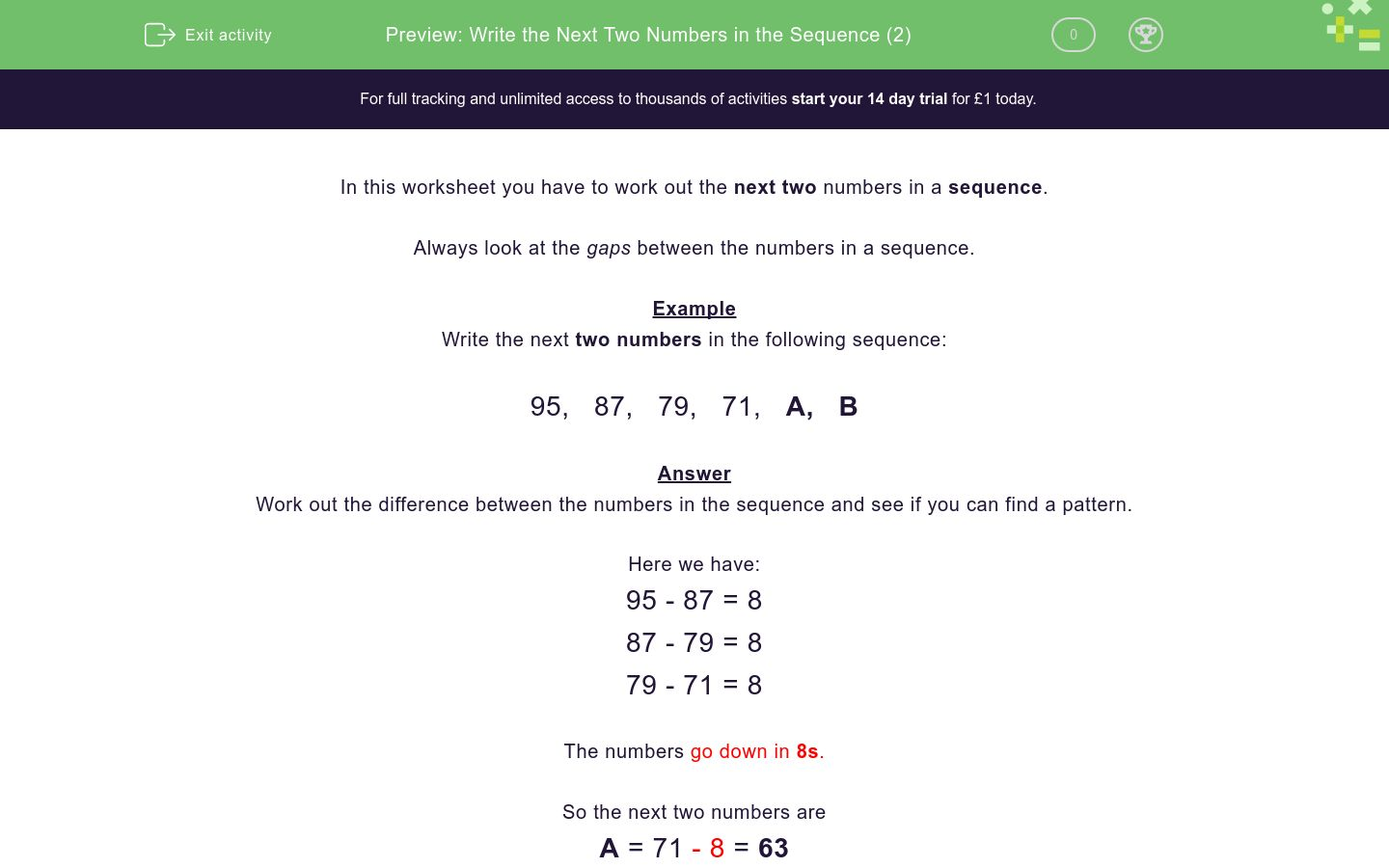# Write the Next Two Numbers in the Sequence (2)

In this worksheet, students learn about number sequences by identifying the difference between successive terms.Key stage:  KS 2

Curriculum topic:   Number: Number and Place Value

Curriculum subtopic:   Solve Number Problems to 1000

Difficulty level:#### Worksheet Overview

In this worksheet you have to work out the next two numbers in a sequence.

Always look at the gaps between the numbers in a sequence.

Example

Write the next two numbers in the following sequence:

95,   87,   79,   71,   A,   B

Work out the difference between the numbers in the sequence and see if you can find a pattern.

Here we have:

95 - 87 = 8

87 - 79 = 8

79 - 71 = 8

The numbers go down in 8s.

So the next two numbers are

A = 71 - 8 = 63

B = 63 - 8 = 55